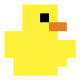1.Jan

Using tocloft and etoolbox, the header of fancyhdr disappears on the TOC page. Using [title]{tocloft} it returns, but the spacing is like without using tocloft and etoolbox. Any idea?

•Hi Jan,

I tried to reproduce what you describe, without success. Please send a minimal working example similar to the code below to illustrate your problem. Thanks, Tom.

\documentclass[12pt]{report}
\usepackage{fancyhdr, blindtext}
\usepackage{etoolbox, tocloft}
\preto\figure{%
}
\pagestyle{fancy}
\begin{document}
\tableofcontents\clearpage
\listoffigures\clearpage
\chapter{Dummy chapter one}
\Blindtext
\begin{figure}[ht]\centering\rule{0.5\textwidth}{0.3\textwidth}\caption{Dummy figure one}\end{figure}
\begin{figure}[ht]\centering\rule{0.5\textwidth}{0.3\textwidth}\caption{Dummy figure two}\end{figure}
\chapter{Dummy chapter two}
\begin{figure}[ht]\centering\rule{0.5\textwidth}{0.3\textwidth}\caption{Dummy figure three}\end{figure}
\begin{figure}[ht]\centering\rule{0.5\textwidth}{0.3\textwidth}\caption{Dummy figure four}\end{figure}
\Blindtext
\end{document}
2.rDuck

Hi there, excellent guide, however I have been unable to get the chapter headings in list of figures to work while using the chemstyle package, loading order doesn’t seem to matter either. and i cant see any problems in the log file, but the chapters simply disappear when i run this package, do you know why? I included a minimal (non) working example below.

%!TEX TS-program = lualatex
\documentclass[12pt]{report}
\usepackage{chemstyle}
\usepackage{graphicx}
\usepackage{etoolbox, tocloft}
\preto\figure{%
}

\begin{document}
\tableofcontents
\listoffigures
\chapter{Introduction}
\section{Background}
\begin{figure}
\centering
\includegraphics[height=23mm]{Test.png}
\caption{Test}
\label{Test}
\end{figure}
\end{document}
•Hi!

I believe that’s because the package or one of its dependencies redefines the list of figure using hooks (\AtEndOfPackage and \AtBeginDocument). It works if you move \preto... further down. Place it just after \begin{document}.

Tom

•rDuck

Awesome! worked like a charm, thank you so much!

•You are very welcome! Tom

3.Reity

YES, YES AND YES…. thank u… the list of figures changes compiled PERFECTLY… at the first shot… and my thesis was already finished… I just added the commands:

\usepackage{etoolbox, tocloft}
\preto\figure{%
}
•tom

Great, thanks for the feedback and all the best with your thesis! Tom

4.Amsul

Thanks for this Tom, I had a quick question, could you tell me instead of just chapter 1 in the list of figures, I want the whole chapter title how I would go about doing that?

•tom

Hi Amsul,

Thanks for the question. Here’s how to produce the name of the chapter in addition to the number.

\documentclass[11pt]{report}
\let\Chaptermark\chaptermark
\def\chaptermark#1{\def\Chaptername{#1}\Chaptermark{#1}}
\usepackage{etoolbox, tocloft}
\preto\figure{%
}
\begin{document}
\tableofcontents
\listoffigures
\chapter{Some chapter}
\begin{figure}[ht]
\centering
\rule{0.5\linewidth}{0.5\linewidth}
\caption{Some caption}
\label{fig:def}
\end{figure}
\end{document}
5.Amsul

Thanks for the reply Tom. I ran into a small problem, with the “chaptername” ‘s c captialized, it would not work, and kept giving me an error. When I made it smaller case, it compiled However instead of the actual chapter name it just kept giving me chapter 1 chapter. Any ideas as to what might be going on?

•tom

You might be using a package that conflicts with this code. Also, \chaptername doesn’t work, because it is reserved for ‘Chapter’. Actually, I should have used the command instead of the word (see code below). Did you try with another word for \Chaptername, e.g. \chapterdef?

\usepackage{etoolbox, tocloft}
\let\Chaptermark\chaptermark
\def\chaptermark#1{\def\chapterdef{#1}\Chaptermark{#1}}

\preto\figure{%
}

If this doesn’t work, please provide a minimal working example.

Thanks, Tom

6.Amsul

Thanks Tom, I think that might be true, I have way too many packages and renewcommands I had to use. I have had to change a lot of things about the chapter heading so that might be why. I’ll try a few things and if not I will post a minimal working example here. Thanks.

•tom

Great! Good luck and let me know should you get stuck again. Best, Tom

•Amsul

Tom,

I tried doing the same thing with tables as you did with figures by changing lof to lot and figure to table in the code, but for some reason my first table in chapter 2 does not show up under chapter but rather by itself. Might you know a reason this is happening?

•Amsul

Actually, just realized, it is doing so with the figure as well, figure 2.1 show up above chapter 2. for some reason?

•tom

Hi Amsul,

Might be a package that you load, which causes a conflict. Please provide a minimal working example so I can reproduce the problem. Thanks.

Here is a basic MWE that works:

\documentclass{report}
\usepackage{etoolbox, tocloft}
\preto\figure{%
}
\preto\table{%
}
\begin{document}
\listoffigures
\listoftables
\chapter{First}
\chapter{Second}
\begin{figure}[ht]\centering\rule{0.5\textwidth}{0.5\textwidth}\caption{Fig caption}\end{figure}
\begin{table}\centering\begin{tabular}{cc}a&amp;b\\c&amp;d\\\end{tabular}\caption{Tab caption}\end{table}
\end{document}
•Amsul

Got it to work, apparently thee was some spacing issue

•tom

Great, thanks for the feedback!

7.I have also tried it. It works flawlessly with the table of contents, but It makes weird things with the list of figures and the list of tables. Sometimes “Chapter #” appears after the item it corresponds, and also sometimes “Chapter #” gets printed twice…

•tom

Would you mind sending a minimal working example to illustrate the issue? I tried and it works for me with figures and tables…

8.MANSI CHAHAL

Sir, how can I modify the default top margin in table of content page and some other pages like list of figures?

•Hi there,

Standard chapters are used to generate these lists. You could temporarily change the chapter margins. See here for more details.

HTH, Tom